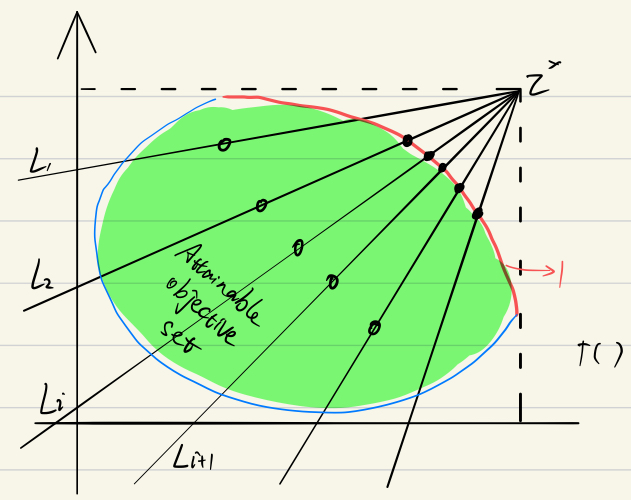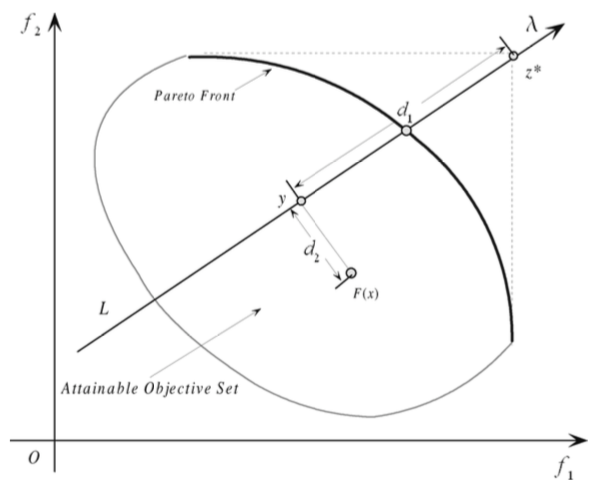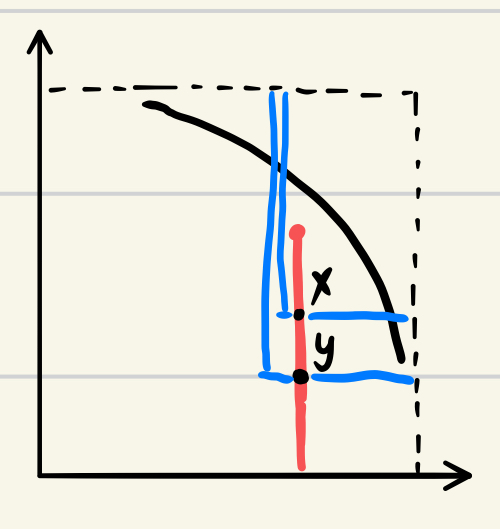MOEA/D 简介

# MOEA/D: A Multiobjective Evolutionary Algorithm Based on Decomposition

## I. Introduction

### A. Basic Definition

• MOP 定义: $maximize\ F(x)=(f_1(x),f_2(x),\cdots,f_m(x))^T\ subjuect\ to\ x\in\Omega$ (1)
• $\Omega$ 定义: 决策空间 decision space，$F:\Omega\rightarrow R^m$
• $R^m$ 定义: objective space
• $\{F(x)|x\in\Omega\}$: attainable objective set
• 限制：对于 $x\in R^n,\Omega=\{x\in R^n|h_j(x)\leq0,j=1,\cdots m\}$, $h_j$ are continuous function，这使得 $x$ 的定义域被限制在一定范围，此时前面那个方程被成为一个连续多目标优化问题。
• Domination: $\forall i\in \{1,\cdots,m\},u_i\geq v_i$ and $\exist j\in \{1,\cdots,m\},u_i> v_i$，即全部大于等于，并至少有一个 index 大于的时候，称其为 $u_i$ dominates $v_i$
• 对于一个点 $x$ 如果 $F(x)$ 在目标域内没有被 dominated，其 $x$ 被称为 Pareto optimal，$F(x)$ 被称为 Pareto optimal vector
• Pareto set (PS): all $x$ which is Pareto optimal point
• Pareto front (PF): all $F(x)$ which is Pareto optimal vector

### B. Current Algorithm Analysis

It is well-known that a Pareto optimal solution to a MOP, under mild conditions, could be an optimal solution of a scalar optimization problem in which the objective is an aggregation of all the $f_i$’s. Therefore, approximation of the PF can be decomposed into a number of scalar objective optimization subproblems. This is a basic idea behind many traditional mathematical programming methods for approximating the PF. Several methods for constructing aggregation functions can be found in
the literature (e.g., ). The most popular ones among them include the weighted sum approach and Tchebycheff approach. Recently, the boundary intersection methods have also attracted a lot of attention –.

• 目前主流的 MOEAs 并没有 decomposition 参与，它们把 MOP 视为一个整体来解决，亦不会把任何一个单独的解与标量优化问题相关联。

• 一般有一个 objective function 来衡量所有解最后只能得到一个最优值。

• Domination 不会在目标空间中定义一个完整的解的顺序，而且 MOEA 主要负责产生尽量离散的 Pareto optimal point 以代表整个 PF。所以，传统的 selection operator 不能直接在 non-decomposition MOEA 中使用。

• However, domination cannot provide a full ranking among all the solutions. (Engin Ufuk Ergul & Ilyas Eminoglu (2014) DOPGA)

• TPLS 会考虑一组 scalar optimization 问题，在这些问题中，目标是在 MOP 考虑下的一个聚类，一个 scalar optimization 算法会基于聚类的系数以一个顺序应用到这些问题上，上一个问题的解会被获取过来以作为下一个问题的起点，尽管他的聚类目标与之前的那个只有微小的区别。
• MOGLS 会同时优化所有聚类使用 weighted sum approach 或者 Tchebycheff approach，在每次迭代，它会优化一个随机生成的聚类目标

### C. MOEA/D

• 会把一个 MOP 分解成 N 个标量优化子问题
• 演化一个人口基数的解集以同时解决这些子问题
• 在每一代，人口都是由每个子问题最优的解组合而成
• 子问题之间的邻居关系是基于他们聚类参数向量之间的距离决定的
• 两个邻居子问题的最优解应该是十分相似的
• 每个子问题在 MOEA/D 的优化过程中，只会使用其邻居的信息

### D. MOEA/D features

• MOEA/D 以一个很有效率的方式把 decomposition approaches 引入多目标演化计算。而分解法，通常是在编程数学社区被发展过后，可以很好的纳入 MOEA/D 框架中的演化算法以解决 MOP
• MOEA/D 优化 N 个标量优化问题，而不是把 MOP 作为一个整体去解决，像 fitness assignment 或者 diversity maintenance 会在 MOEAS 上产生困难的这些情况，在 MOEA/D 框架下能更容易去控制
• MOEA/D每一代的计算复杂度都低于 NSGA-II 和 MOGLS
• 目标正则化的技巧可以纳入算法之中，以解决一些不同规模(disparately scaled)的目标
• 每个解都关联了一个标量优化问题，所以可以很自然地使用标量优化方法。相对应的，非分解的 MOEAs 并不能很容易的用到 scalar optimization methods 的好处

## II. Decomposition of Multiobjective Optimization

### A. Weighted Sum Approach

DEF:

• weight vector:

$\pmb\lambda=(\lambda_1,\cdots,\lambda_m)^T,\ \lambda_i\geq0,\ \sum_{i=1}^{m} \lambda_i=1$

• 标量优化问题 (2)

$maximize\ g^{ws}(x|\pmb\lambda)=\sum_{i=1}^{m} \lambda_if_i(x)$

### B. Tchebycheff Approach

DEF:

• 标量优化问题 (3)

$minimize\ g^{te}(x|\pmb\lambda,z^*)=\max_{1\leq i\leq m} \{\lambda_i|f_i(x)-z_i^*\}$

• reference point

\begin{aligned} z^*&=(z_1^*,\cdots,z_m^*)^T \\ z_i^*&=max\{f_i(x)|x\in \Omega\} \end{aligned}

### C. Boundary Intersection (BI) Approach

Normal Constraint Method 。一般情况下，PF 在 maximize 下是最右上方的边界(minimize 是左下方)。通常 BI 是要找到一组线与 PF 的交点，所以如果这组线很均匀的分布的话，这些个交点就可以很好的代表这个 PF。

DEF:

\begin{aligned} minimize\ g^{bi}(x|\pmb\lambda,z^*)&=d\ \ \ \ \ \ \ \ \ \ \ \ \ \ \ \ \ \ \ \ \ \ \ \ \ \ \ \ \ \ \ \ \ \ \ \ \ \ (4)\\ subject\ to\ z^*-F(x)&=d\pmb\lambda \\ x &\in \Omega \end{aligned}DEF:

\begin{aligned} minimize\ g^{bip}(x|\pmb\lambda,z^*)&=d_1+\theta d_2\ \ \ \ \ \ \ \ \ \ \ \ \ \ \ \ \ \ \ \ \ \ \ \ \ \ \ \ \ \ \ \ \ (5)\\ subject\ to\ x &\in \Omega \end{aligned}

where

\begin{aligned} d_1&=\frac{||(z^*-F(x))^T\pmb\lambda||}{||\pmb\lambda||} \\ and\ d_2&=||F(x)-(z^*-d_1\pmb\lambda)|| \end{aligned}PBI 的好处都有啥（与 TE 相比）：

• PBI 的解的分布比 TE 更加均匀，尤其是在 weight vector 不是特别多的时候
• TE 在 x dominate y 的情况下，也会有 $g^{te}(x|\pmb\lambda,z^*)=g^{te}(y|\pmb\lambda,z^*)$，如下图## III. MOEA/D

### A. General Framework

#### 预设

$\pmb\lambda^1,…,\pmb\lambda^N$ 是均匀分布的权重向量

$z^*$ 是 ref point

$minimize\ g^{te}(x|\pmb\lambda^j,z^*)=\max_{1\leq i\leq m} \{\lambda_i^j|f_i(x)-z_i^*\}$

• 具有 $N$ 个解 $x^1, …, x^N$ 的总人口，$x^i$ 对应的是第 $i$ 个子问题的解
• $FV^i,i=1,…,N$ 是对于第 $i$ 个解的 F value
• $z=(z_1,…,z_m)^T$ ，这里面 $z_i$ 是对于目标 $f_i$ 目前所能达到的最优的值
• 还有一个 external population (EP) 来存放所有在搜索中发现的一些非统治地位的解，也就是最终结果

#### 算法

##### a. INPUT
1. MOP
2. 一个停止 criteria
3. $N$
4. $N$ 个均匀分布的权重向量 $\pmb\lambda^1,…,\pmb\lambda^N$
5. $T$: 邻居的数量

EP

##### c. STEP

Step 1) Initialization
Step 1.1) $EP=\empty$
Step 1.2) 计算任意两个权重向量之间的欧式空间距离，算出 $T$ 个邻居 $B(i)=\{i_1,…,i_T\}$，然后 $\pmb\lambda^{i_1},…,\pmb\lambda^{i_T}$ 即为那些邻居
Step 1.3) 根据题设生成基础人口 $x^1, …, x^N$，设置 $FV^i=F(x_i)$
Step 1.4) 初始化 $z$

Step 2) Update
For i = 1,…,N Do
Step 2.1 繁殖) 从邻居 $B(i)$ 中随机选择两个 $k,l$$x^k,x^l$ 由基因操作子来产生子代 $y$
Step 2.2 增强)$y$ 使用一个题设的修复/增强的启发式方法，产生 $y'$
Step 2.3 更新$\pmb z$)$y'$ 来更新 $z$
Step 1.4 更新邻居) 对于邻居 $B(i)$ 中的每个 $j$ ，如果 $g^{te}(y'|\pmb\lambda^j,z) \leq g^{te}(x^j|\pmb\lambda^j,z)$，则 $x^j=y',FV^j=F(y')$
Step 1.4 更新 EP) 移除 EP 中所有被 $F(y')$ dominate 的解，然后把 $F(y')$ 加入

Step 3) Stopping 如果满足停止条件，输出 EP，否则重复 STEP2

### B. Discussions

1. 为什么只考虑有限数量个子问题

MOGLS 就是每代随机生成新的权重向量，要知道我们只需要有限个均匀分布的解来表示 PF 就可以了，所以这个方法既现实又合理，可节约计算资源

2. 多样化

Section I 中说过 MOEA 需要维持一个多样化的人口来产生一系列具有代表性的解。NSGA-II and SPEA-II use crowding distances among the solutions in their selection to maintain diversity. 但那些算法并不是很容易就能产生一个均匀分布的目标向量。MOEA/D 中每个子问题和每个人口相关联，而且权重向量也是选取的很恰当，所以可以保证解的均匀分布性

3. Mating Restriction and the Role of T in MOEA/D

一个新解的产生仅基于邻居中的父母，所以如果邻居太小，子代会与父母具有极高的相似性，不利于人口多样性与探索解的新的区域，但如果邻居太大，父母又是不能很好的去优化本子问题，所以 exploration ability 也会变得很差。

### C. Variants of MOEA/D

1. 可以用不同的 decomposition method
2. 返回结果的时候可以使用 final population，如果 EP 没有被维护的话。(以及再加上 EP size 的限制) 
3. cMOGA 也可以被视为使用了 decomposition 但并不能维持一个最佳解集 

## Other

• IV. COMPARISON WITH MOGLS
• A. 什么是 MOGLS
• B. MOEA/D 和 MOGLS 的时空复杂性的对比
|||我觉得这部分我还得在暑假看一下计算理论的书才能知道详细步骤，而且毕竟也是要考的
• C. 什么是多目标 01背包问题(MOKP)
• D. MOEA/D 和 MOGLS 在 MOKP 上的实现方法，算法步骤
• E. 实验参数设置
• F. 然后就是一堆实验结果
• V. MOEA/D 与 NSGA-II 在连续性 MOP 上的对比
说到连续性，就是那些 continuous function，通常只是一个简单的函数而已
• A. 会有 Test Suites: ZDT, DTLZ 等跑分函数
• B. 什么是 NSGA-II，(步骤是真的少，那篇文读了才开头)
• C. 使用 MOEA/D 的变体
• D. 两者复杂性分析
• E. 实验参数设置
• F. 实验结果
• G. MOEA/D 扩展
• VI. 结论
• 居然姚新被 Acknowledge 了，zhou和lucas也被提及了，zqf是真的巨

 K. Miettinen*, Nonlinear Multiobjective Optimization*. Norwell, MA: Kluwer, 1999.

 I. Das and J. E. Dennis, “Normal-bounday intersection: A new method for generating Pareto optimal points in multicriteria optimization prob- lems,” SIAM J. Optim., vol. 8, no. 3, pp. 631–657, Aug. 1998.

 A.Messac,A.Ismail-Yahaya,andC.Mattson,“Thenormalizednormal constraint method for generating the Pareto frontier,” Struct Multidisc. Optim., vol. 25, pp. 86–98, 2003.

 J. D. Knowles and D. Corne, “Properties of an adaptive archiving algo- rithm for storing nondominated vectors,” IEEE Trans. Evol. Comput., vol. 7, no. 2, pp. 100–116, Apr. 2003.

 T. Murata, H. Ishibuchi, and M. Gen*,* “Specification of Genetic Search Direction in Cellular Multiobjective Genetic Algorithm,” in Evolutionary Multicriterion Optimization. Berlin, Germany: Springer-Verlag, 2001, LNCS1993, pp. 82–95.

Author: Hackroid